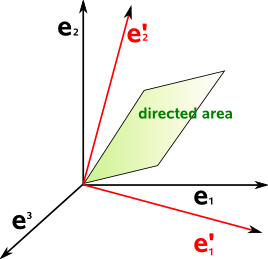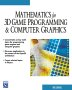# Maths - Vector Related Ideas - Bivectors and Covectors

On these pages we look at Bivectors and Covectors, concepts which are similar to vectors and related to them. In both cases there is a sort of duality with vector space. These two entities arise out of different concepts and although they are different they may be related.

## Bivectors

Goto this page to read about bivectors, this comes from the ideas of Clifford algebras and Geometric Algebra so see these pages for a more in depth look at these concepts.

## Covectors

Goto this page to read about covectors, this comes from the ideas of Tensor Algebra so see these pages for a more in depth look at these concepts.

## Bivectors and Covectors in 3 Dimensions

in 3 dimensions bivectors and covectors are related and effectively represent the same concept. We can think of the bivector as being made from the reciprocal basis.

So if we have a vector:

v= A1 e1+ A2 e2+ A3 e3

where:

• e1,e2,e3 = basis vectors

Then if we rotate the basis (e1,e2,e3) then we must rotate the components (A1,A2,A3) in the opposite direction to represent the same vector.

If we now take a bivector:

v1× v2= B1 e2 e3 + B2 e3 e1 + B3 e1 e2

Then if we rotate the basis (e1,e2,e3) then how must we rotate the components (B1,B2,B3) to represent the same bivector?

To illustrate this imagine a directed area (bivector) in the e1e2 plane:Then imagine that we rotate the basis vectors e1 and e2 around the e3 basis to form e'1 and e'2.

To represent the same parallelogram then the components of the vectors must be rotated in the reverse direction.

However to represent the same directed area then the components of the vectors don't need to be changed, the directed area will change with changes in the normal direction.

So in 3 dimensions there is a duality between vector basis which is contravarient and bivector basis which is covariant.

vector basis

contravarient

bivector basis

covariant

e1 e2 e3
e2 e3 e1
e3 e1 e2

### 'n' Dimensions

The above only applies in 3 dimensions, in 4 dimensions then bivectors are 6 dimensional so we cant have such a duality, in 4 dimensions there is a duality between vectors and trivectors.

In 'n' dimensions there is a duality between the grades on opposite sides of the binomial triangle. however I have not checked if there is always a parallel contravarient/covariant duality.

## Duality

 metadata block see also: Correspondence about this page Book Shop - Further reading. Where I can, I have put links to Amazon for books that are relevant to the subject, click on the appropriate country flag to get more details of the book or to buy it from them.Mathematics for 3D game Programming - Includes introduction to Vectors, Matrices, Transforms and Trigonometry. (But no euler angles or quaternions). Also includes ray tracing and some linear & rotational physics also collision detection (but not collision response).Covers VRML 2 (but not the upcoming X3D standard). A good introduction to all VRML2 node types and how to build them. Other Math Books Specific to this page here:

This site may have errors. Don't use for critical systems.

Copyright (c) 1998-2021 Martin John Baker - All rights reserved - privacy policy.